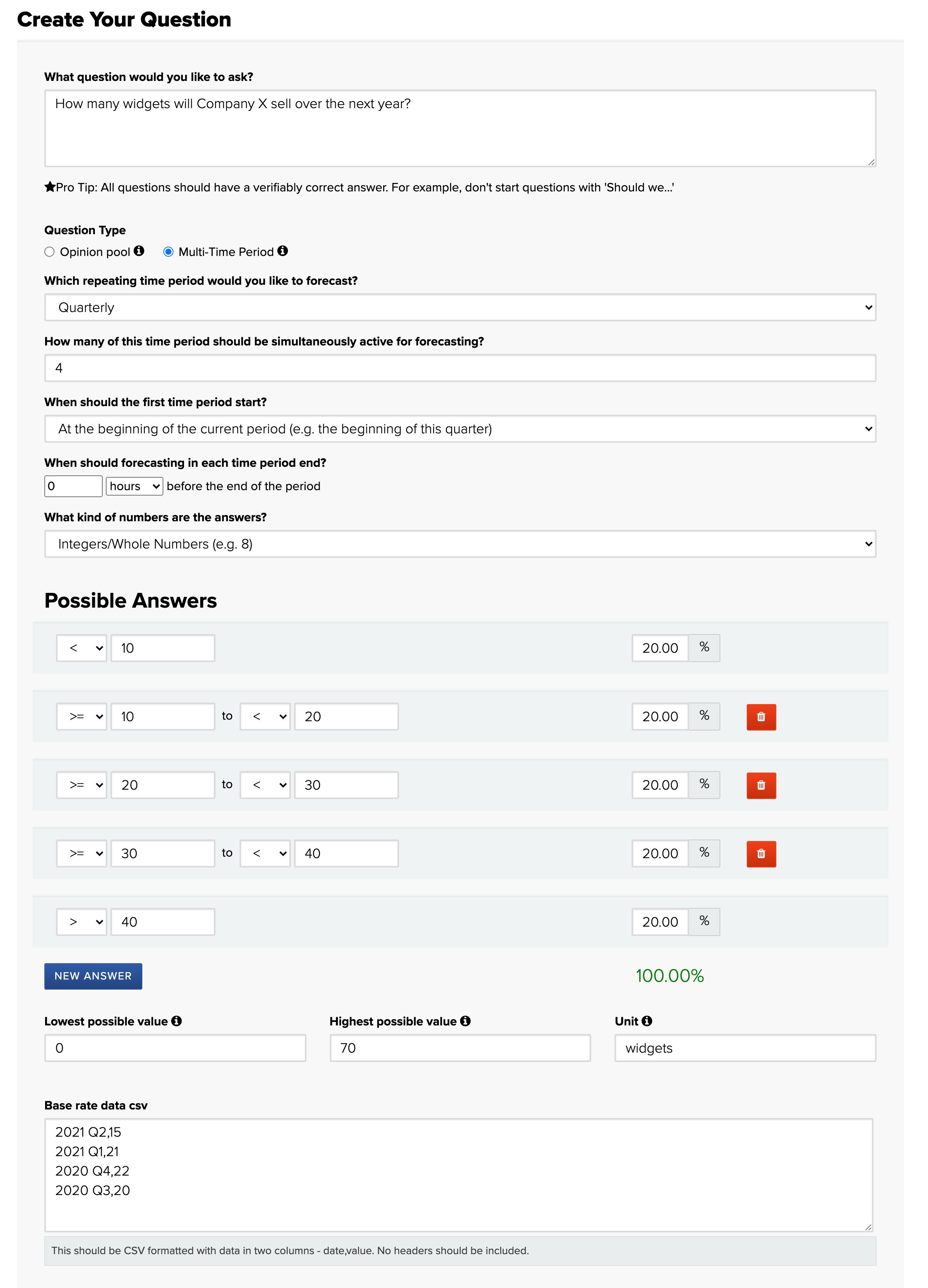Cultivate Labs Support Article

## Creating a Multi-time Period Question

For an introduction to multi-time period questions, you can read our blog post here

To publish a multi-time period question:

• Go to the Admin "home"
• On the left-hand navigation bar, select "Questions".
• Click the "create a new question" button
• Fill out your question text
• Select "Multi-Time Period" as the Question Type

Once you've completed those steps, you'll see a form that looks something like:Below is a description of each of the available fields:

• Which repeating time period would you like to forecast? - This field determines the time period being forecasted. For example, if you want to forecast quarterly earnings for a company, you would select "Quarterly" for this field.
• How many of this time period should be simultaneously active for forecasting? - In these questions, multiple time periods can be open for simultaneous forecasting (hence the name, multi-time period). So if you want to forecast quarterly earnings for a year into the future, you could enter 4 in this field and users would be solicited to enter forecasts for each of the next 4 quarters. If you wanted to forecast two years into the future, you could enter 8 quarters.
• When should the first time period start? - This field determines when the first forecasting period will begin. If you're forecasting something quarterly and select "At the beginning of the current period," then the first forecasting period would be the quarter of the year that you're currently in. If you select "At the start of the next period," then the first forecasting period would be the beginning of the next quarter.
• When should forecasting in each time period end? - If you would like forecasting to end before the end of the time period, you can enter a value in this field. For example, if you were forecasting quarterly and want forecasting to end a month before the end of the quarter, you would enter "1 month" in this field.
• What kind of numbers are the answers? - All multi-time period questions are forecasting a numerical value. This field allows you to specify if that value is an integer (e.g. number of widgets sold) or a decimal (e.g. dollars of revenue). This is then used to validate the numerical bounds of the possible answers, ensuring there are no gaps between the answers.
• Possible Answers - While forecasters will not see these values, all questions must fit into a format that can be Brier scored, so an admin must define underlying answer buckets for the question.  User's forecasts will then be automatically partitioned into these buckets for scoring. Ideally, the bounds of the answer buckets will be defined such that the answers' probabilities will roughly fit a normal distribution.
• Lowest possible value - This value defines the lowest possible value that a forecaster will be able to enter in the forecasting interface.
• Highest possible value -This value defines the highest possible value that a forecaster will be able to enter in the forecasting interface.
• Unit - This field is used as a label when displaying the quantity being forecasted (e.g. "widgets" or "dollars").
• Base rate data csv - When entered, this field will be used to display historical values for the given question. If you're forecasting quarterly earnings, you can use this field to provide forecasters with information about actuals for past quarters. If data is not available, leave this field blank. It should be entered one time period per line and formatted as time-period,value (e.g. "2021 Q2,15").

#### Still have questions?

 More support articles support@cultivatelabs.com